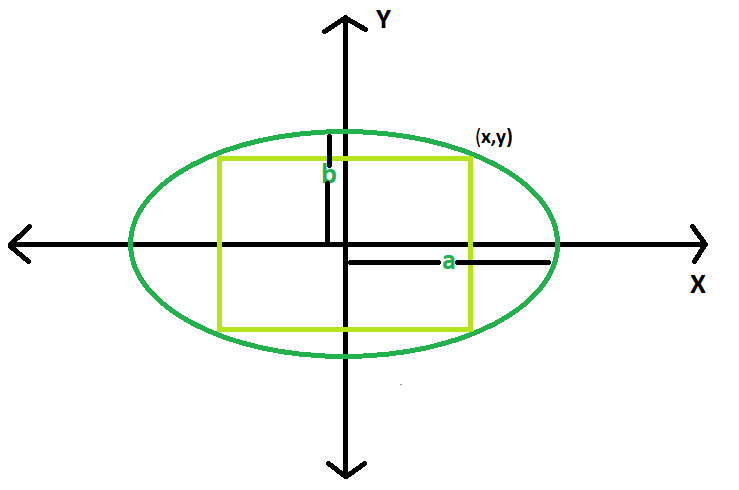GFG App
Open AppBrowser
Continue

# Area of Largest rectangle that can be inscribed in an Ellipse

Given an ellipse, with major axis length 2a & 2b. The task is to find the area of the largest rectangle that can be inscribed in it.
Examples

```Input: a = 4, b = 3
Output: 24

Input: a = 10, b = 8
Output: 160```Approach
Let the upper right corner of the rectangle has co-ordinates (x, y)
Then the area of rectangle, A = 4*x*y.
Now,

Equation of ellipse, (x2/a2) + (y2/b2) = 1
Thinking of the area as a function of x, we have
dA/dx = 4xdy/dx + 4y
Differentiating equation of ellipse with respect to x, we have
2x/a2 + (2y/b2)dy/dx = 0,
so,
dy/dx = -b2x/a2y
and
Setting this to 0 and simplifying, we have y2 = b2x2/a2.
From equation of ellipse we know that,
y2=b2 – b2x2/a2
Thus, y2=b2 – y2, 2y2=b2, and y2b2 = 1/2
Clearly, then, x2a2 = 1/2 as well, and the area is maximized when
x= a/√2 and y=b/√2
So the maximum area, Amax = 2ab

Below is the implementation of the above approach:

## C++

 `// C++ Program to find the biggest rectangle` `// which can be inscribed within the ellipse` `#include ` `using` `namespace` `std;`   `// Function to find the area` `// of the rectangle` `float` `rectanglearea(``float` `a, ``float` `b)` `{`   `    ``// a and b cannot be negative` `    ``if` `(a < 0 || b < 0)` `        ``return` `-1;`   `    ``// area of the rectangle` `    ``return` `2 * a * b;` `}`   `// Driver code` `int` `main()` `{` `    ``float` `a = 10, b = 8;` `    ``cout << rectanglearea(a, b) << endl;` `    ``return` `0;` `}`

## Java

 `// Java Program to find the biggest rectangle` `// which can be inscribed within the ellipse`   `import` `java.util.*;` `import` `java.lang.*;` `import` `java.io.*;`   `class` `GFG{ ` `// Function to find the area` `// of the rectangle` `static` `float` `rectanglearea(``float` `a, ``float` `b)` `{` ` `  `    ``// a and b cannot be negative` `    ``if` `(a < ``0` `|| b < ``0``)` `        ``return` `-``1``;` ` `  `    ``// area of the rectangle` `    ``return` `2` `* a * b;` `}` ` `  `// Driver code` `public` `static` `void` `main(String args[])` `{` `    ``float` `a = ``10``, b = ``8``;` `    ``System.out.println(rectanglearea(a, b));` `}` `}`

## Python 3

 `# Python 3 Program to find the biggest rectangle ` `# which can be inscribed within the ellipse `   `#  Function to find the area ` `# of the rectangle ` `def` `rectanglearea(a, b) :`   `    ``# a and b cannot be negative ` `    ``if` `a < ``0` `or` `b < ``0` `:` `        ``return` `-``1`   `    ``# area of the rectangle` `    ``return` `2` `*` `a ``*` `b` ` `    `# Driver code     ` `if` `__name__ ``=``=` `"__main__"` `:`   `    ``a, b ``=` `10``, ``8` `    ``print``(rectanglearea(a, b))`     `# This code is contributed by ANKITRAI1`

## C#

 `// C# Program to find the` `// biggest rectangle which ` `// can be inscribed within` `// the ellipse` `using` `System;`   `class` `GFG` `{ ` `// Function to find the area` `// of the rectangle` `static` `float` `rectanglearea(``float` `a, ` `                           ``float` `b)` `{`   `    ``// a and b cannot be negative` `    ``if` `(a < 0 || b < 0)` `        ``return` `-1;`   `    ``// area of the rectangle` `    ``return` `2 * a * b;` `}`   `// Driver code` `public` `static` `void` `Main()` `{` `    ``float` `a = 10, b = 8;` `    ``Console.WriteLine(rectanglearea(a, b));` `}` `}`   `// This code is contributed ` `// by inder_verma`

## PHP

 ``

## Javascript

 ``

Output

`160`

Time Complexity: O(1)
Auxiliary Space: O(1)

My Personal Notes arrow_drop_up